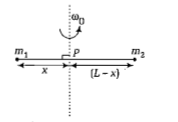A solid sphere of mass m and radius R is rotating about its diameter. A soild cyclinder of the same mass and same radius is also rotating about its geometrical axis with an angular speed twice that of the sphere. The ratio of their kinetic energies of rotation  will be

(a) 2:3

(b) 1:5

(c) 1:4

(d) 3:1

Concept Questions :-

Moment of inertia
High Yielding Test Series + Question Bank - NEET 2020

Difficulty Level:

A light rod of length l has two masses  attached to its two ends. The moment of inertia of the system about an axis perpendicular to the rod and passing through the centre of mass is

(a) $\frac{{m}_{1}{m}_{2}}{{m}_{1}+{m}_{2}}{l}^{2}$

(b)$\frac{{m}_{1}+{m}_{2}}{\sqrt{{m}_{1}{m}_{2}{l}^{2}}}{l}^{2}$

(c)$\left({m}_{1}+{m}_{2}\right){l}^{2}$

(d) $\sqrt{{m}_{1}{m}_{2}{l}^{2}}$

Concept Questions :-

Moment of inertia
High Yielding Test Series + Question Bank - NEET 2020

Difficulty Level:

From a disc of radius R and mass M, a circular hole of diameter R, whose rim passes through the centre is cut. What is the moment of inertia of the remaining part of the disc about a perpendicular axis, passing through the centre ?

(a)         (b)

(c)           (d)

Concept Questions :-

Moment of inertia
High Yielding Test Series + Question Bank - NEET 2020

Difficulty Level:

A rod of weight w is supported by two parallel knife edges A and B and is in equilibrium in a horizontal position. The knives are at a distance d from each other. The centre of mass of the rod is at distance x from A. The normal reaction on A is

(a)$\frac{\mathrm{wx}}{\mathrm{d}}$

(b)$\frac{\mathrm{wd}}{\mathrm{x}}$

(c)w(d-x)/x

(d)$\frac{w\left(d-x\right)}{d}$

Concept Questions :-

Torque
High Yielding Test Series + Question Bank - NEET 2020

Difficulty Level:

Three identical spherical shells, each of mass m and radius r are placed as shown in figure. Consider an axis XX’, which is touching to two shells and passing through diameter of third shell. Moment of inertia of the system consisting of these three spherical shells about XX' axis is1.$\frac{11}{5}$mr2

2. 3mr2

3. $\frac{16}{5}$mr2

4. 4mr2

Concept Questions :-

Moment of inertia
High Yielding Test Series + Question Bank - NEET 2020

Difficulty Level:

Point masses m1 and m2 are placed at the opposite ends of a rigid rod of length l and negligible mass. The rod is to be set rotating about an axis perpendicular to it. The position of point P on this rod through which the axis should pass, so that the work required to set the rod rotating with angular velocity ωo is minimum, is given by(a)$x=\frac{{m}_{1}L}{{m}_{1}}$$\frac{}{+{m}_{2}}$
(b)$x=\frac{{m}_{1}}{{m}_{2}}L$
(c)$x=\frac{{m}_{2}}{{m}_{1}}L$
(d)$x=\frac{{m}_{2}L}{{m}_{1}+{m}_{2}}$

Concept Questions :-

Moment of inertia
High Yielding Test Series + Question Bank - NEET 2020

Difficulty Level:

Two particles A and B. move with constant velocities v1 and v2. At the initial moment, their position vectors are ${r}_{1}$ and ${r}_{2}$ respectively. The condition for particles A and B for their collision is-

(a) $\frac{{r}_{1}-{r}_{2}}{\left|{r}_{1}-{r}_{2}\right|}=\frac{{v}_{2}-{v}_{1}}{\left|{v}_{2}-{v}_{1}\right|}$

(b) ${r}_{1}·{v}_{1}={r}_{2}·{v}_{2}$

(c) ${r}_{1}×{v}_{1}={r}_{2}×{v}_{2}$

(d) r1-r2=v1-v2

Concept Questions :-

Linear momentum
High Yielding Test Series + Question Bank - NEET 2020

Difficulty Level:

A force F=$a\stackrel{^}{i}$$+3\stackrel{^}{j}$$+6\stackrel{^}{k}$ is acting at a point r=$2\stackrel{^}{i}$-$6\stackrel{^}{j}$-12$\stackrel{^}{k}$. The value of α for which angular momentum about origin is conserved is

(a) -1

(b) 2

(c) zero

(d) 1

Concept Questions :-

Angular momentum
High Yielding Test Series + Question Bank - NEET 2020

Difficulty Level:

On a frictionless surface, a block of mass M moving at speed v collides elastically with another block of same mass M which is initially at rest. After collision the first block moves at an angle θ to its initial direction and has a speed v/3. The second block's speed after the collision is

(a)2√2/3v

(b)3/4v

(c)3/√2v

(d)√3/2v

Concept Questions :-

Linear momentum
High Yielding Test Series + Question Bank - NEET 2020

Difficulty Level:

An automobile moves on a road with a speed of 54 km h-1. The radius of its wheels is 0.45 m and the moment of inertia of the wheel about its axis of rotation is 3 kg m2. If the vehicle is brought to rest in 15 s, the magnitude of average torque transmitted by its brakes to the wheel is

(a)6.66 kgm2s-2

(b)8.58 kgm2s-2

(c)10.86 kgm2s-2

(d)2.86 kgm2s-2

Concept Questions :-

Torque